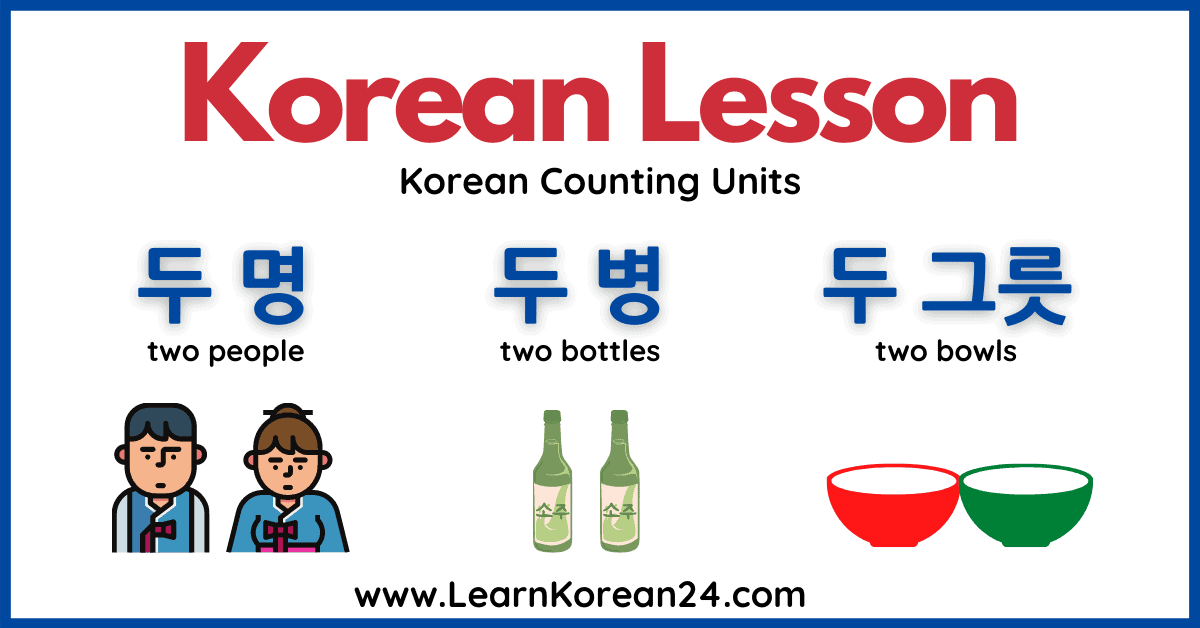# Korean Counting UnitsIn this lesson, you will learn about Korean counting units, and how to ask and answer ‘How many?‘ in Korean. In order to ask and answer ‘How many?’ in Korean, you need to know Korean numbers and Korean counting units. In the previous lesson, you learned Sino Korean numbers and native Korean numbers.

In this lesson, we will teach you how to ask ‘How many?’ in Korean and how to use Korean counting units together with Korean numbers to answer this question. First, let’s look at the basic way to say ‘How many?’ in Korean.

## ‘How Many?’ In Korean

Before we learn about Korean counting units, let’s first look at how to say ‘How many?’ in Korean. In English, the word ‘how’ has different functions. For example, when asking ‘How many?’ , ‘How much?’, ‘How did you do that?’, etc, in English, the word ‘how’ is the same In Korean, however, there are 6 different ways to say ‘How’ in Korean.

In a previous lesson, you learned that ‘How much is it?‘ in Korean is ‘얼마예요?‘. In this sentence, ‘how’ is 얼마 and 예요 is an ending which means ‘is equals to’. When asking ‘How many?’ in Korean, a different word for ‘how’ is used. So, how do you say ‘how many’ in Korean?

The most basic way to ask how many in Korean is 몇 개예요? [myeot gae-ye-yo]. This is made up of (how many) + (a Korean counting unit meaning ‘thing’) + 예요 (ending which means ‘is equals to’).

As this way of saying ‘How many?’ in Korean includes the Korean counting unit , meaning ‘thing’, this literally means ‘How many things?’ or ‘How many are there?’. This can be used in many situations to ask about how many objects there are. Here are some other example sentences using the Korean counting unit :

## Korean Counting Units

The Korean counting unit cannot be used in all situations. There are many different counting units in Korean and if you don’t know a particular counting unit you can usually just use instead. However, you cannot use when referring to people, animals, or actions. Instead, you should use the correct counting unit.

### Common Korean Counting Units

Here are some of the most common Korean counting units:

### Korean Counting Units – Review Quiz

Check how many of these Korean counting units you can remember with this fun review quiz.

Korean Counting Units - Review Quiz

## Counting In Korean

When counting things in Korean and answering the question ‘How many?’, native Korean numbers are used. However, native Korean numbers only go up to 99. If you are counting anything that is more than 99 things, then sino-Korean numbers are used. To review native and sino-Korean numbers, check out our complete guide to numbers in Korean.

Another important thing to remember is that when adding a counting unit to numbers 1,2,3, 4, and 20 the words for these numbers change slightly. Let’s look at some examples:

• ‘One Thing’ in Korean is 하나 (one) + (counting unit meaning ‘thing’) = 한 개 (not 하나 개)
• ‘Two People’ in Korean is (two) + (counting unit for people) = 두 명 (not 둘 명)
• ‘Three Bottles’ in Korean is (three) + (counting unit for bottles) = 세 병 (not 셋 병)
• ‘Four Glasses’ in Korean is (four) + (counting unit for glasses) = 네 잔 (not 넷 잔)
• ‘Twenty Birds’ in Korean is 스물 (twenty) + 마리 (counting unit for birds) = 스무 마리 (not 스물 마리)

For all other native Korean numbers and Sino-Korean numbers for things more than 99, the word doesn’t change. Simply add the counting unit after the number to say how many of that thing there are. Here are some useful example sentences using counting units in Korean. The counting units are in bold.